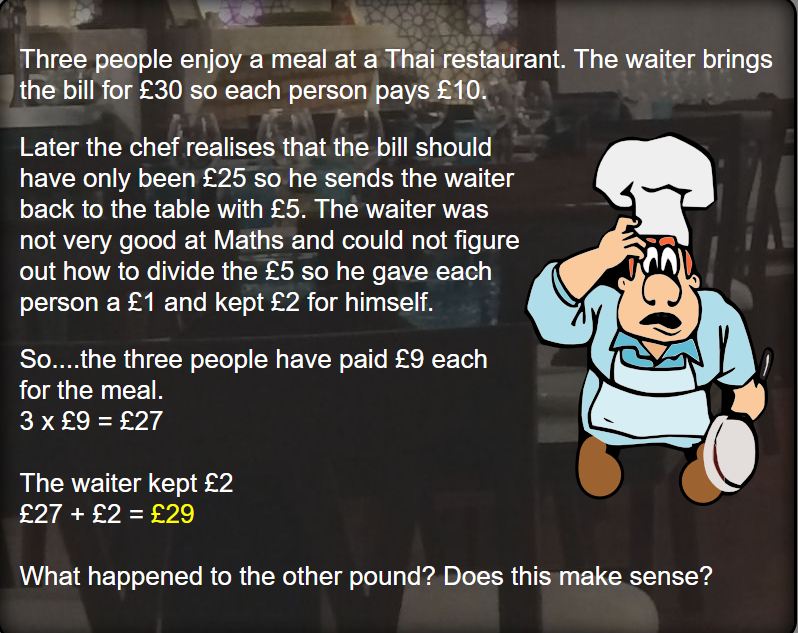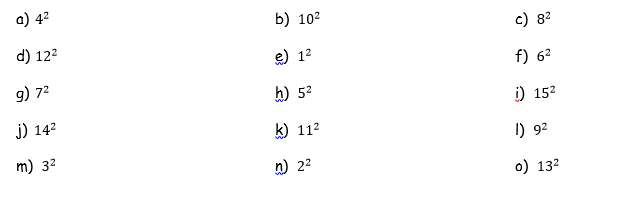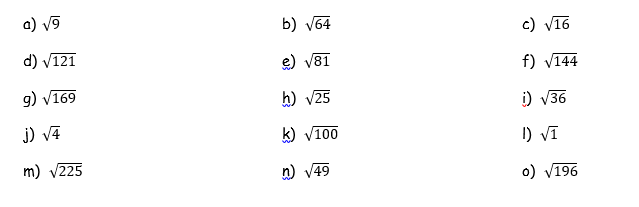# Thursday 18th June – Year 6

Warm up activity:Have a listen to the next chapter of Harry Potter:

Remember these are the login details that you will need:

SPaG:

Complete this lesson to remind yourself of the different ways that you could use commas.

https://www.bbc.co.uk/bitesize/articles/zdy8qnb

Extra task: Make a poster that explains how to use commas. You could focus on one type of comma (i.e. commas in a list) or on all commas. Make it clear and bright. Send them to me as I would like to put them on the blog and up in the Year 6 classroom so other children can use them to help with their writing.

Maths:

Today, we are going to recap some key maths skills: square numbers, square roots and cube numbers.

First up: square numbers.

Can you remember what square numbers are?

If I said 4² what would the answer be?

4² = 16

Remember that this symbol ² is the symbol for squared and it means the number times by itself.

4² =

4 x 4 = 16

6² =

6 x 6 = 36

So 6² = 36

Have a go at these questions.Now let’s look at square roots. They look like this:

√64 (often the little tick at the front has a line that goes across the top of the numbers)

Square root is the opposite of squared numbers i.e.

8² = 64

√64 = 8

You are working out what has been multiplied together to get that answer.

√100 =

For this you would say ‘what has been times by itself to get 100?’

10 x 10 = 100 therefore √100 = 10

Here is another:

√49 =

‘What times by itself = 49?’

7 x 7 = 49 therefore √49 = 7.

Try these questions yourself:Now let’s look at cube numbers. What can you remember?

What is the answer to 4³?

4³ = 64

Can you remember why?

This symbol ³ means cubed. It is when you use the following calculation:

Number multiplied by itself then multiplied by itself again.

4³ =

(4 x 4) x 4 =

(16) x 4 =

16 x 4 = 64

4³ = 64

Let’s try another one:

7³ =

(7 x 7) x 7 =

49 x 7 = 343

7³ = 343

Try these questions now:

a) 3³                              b) 8³                                       c) 2³                            d) 10³

e) 11³                           f) 9³                                        g) 1³                           h) 4³               i) 7³

j) 5³                                k) 12³ l) 15³                          m) 6³                          n) 13³

Finally complete these challenges:Science:

Over the next few weeks, we will be going over some of the scientific knowledge that we have learnt this year and some that you will have learnt in previous years.

This week we will begin with reversible and irreversible changes.

What can you remember?

Vocabulary:

Reversible – able to be changed

Irreversible – unable to be changed

What changes can you think of that are:

– Reversible i.e. water freezes to make ice and can be turned back into water by warming it up.

– Irreversible i.e. egg yolk starts off as a liquid. When it is cooked it becomes a solid and can not be changed back.

Can you think of any other examples?

https://www.bbc.co.uk/bitesize/articles/zndmhg8

Other tasks you could do today:

### 8 thoughts on “Thursday 18th June – Year 6”

•18th June 2020 at 10:00 am

A.16
B.100
C.64
D. 144
E.1
F.36
G.49
H.25
I.225
J.196
K.121
L.81
M.9
N.4
0. 169

•18th June 2020 at 10:11 am

A. 3
B. 8
C. 4
D. 11
E. 9
F. 12
G. 13
H. 5
I. 6
J. 2
K. 10
L. 1
M. 15
N. 7
O. 14

•18th June 2020 at 4:58 pm

Excellent work Emmanuela!!

•18th June 2020 at 11:30 am

•18th June 2020 at 4:58 pm

Great work Ebony!!

•18th June 2020 at 1:38 pm

The warm up was wrong because there were three people not 2 and it said the waiter gave each of them one pound e( which eaquals 3 pound) and kept two pound for himself that all together eaquals 5 pounds add the 25 pounds eaquals 30 so there is no missing pound.

•18th June 2020 at 4:58 pm
•